# Digital Electronics - Combinational Logic Circuits

Exercise : Combinational Logic Circuits - Filling the Blanks
1.

For the XNOR gate truth table shown below, the values for w, x, y, and z are ____, ____, ____, and ____, respectively.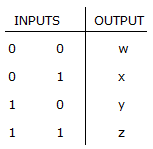1, 0, 0, 1
0, 1, 0, 1
1, 1, 1, 0
1, 0, 0, 0
Explanation:
No answer description is available. Let's discuss.

2.
A half-adder does not have ________.
carry in
carry out
two inputs
all of the above
Explanation:
No answer description is available. Let's discuss.

3.
The equation ________ cannot be further simplified.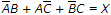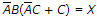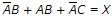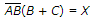Explanation:
No answer description is available. Let's discuss.

4.
________ is a correct combination for an ODD-parity data transmission system.
data = 1101 1011
parity = 1
data = 1101 0010
parity = 0
data = 0001 0101
parity = 1
data = 1010 1111
parity = 0